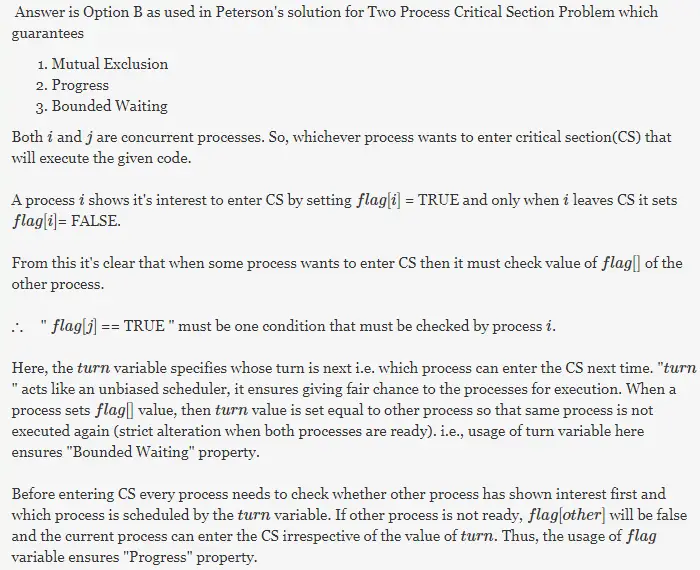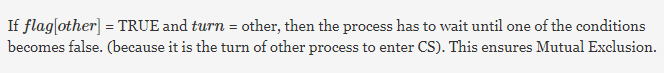# Gate CS-2001 Question Paper With Solutions

Q. 47 Consider Peterson’s algorithm for mutual exclusion between two concurrent processes i and j. The program executed by process is shown below.

```   repeat
flag [i] = true;
turn = j;
while ( P ) do no-op;
Enter critical section, perform actions, then exit critical
section
flag [ i ] = false;
Perform other non-critical section actions.
until false;```

For the program to guarantee mutual exclusion, the predicate P in the while loop should be.

(A) flag[j] = true and turn = i

(B) flag[j] = true and turn = j

(C) flag[i] = true and turn = j

(D) flag[i] = true and turn = i